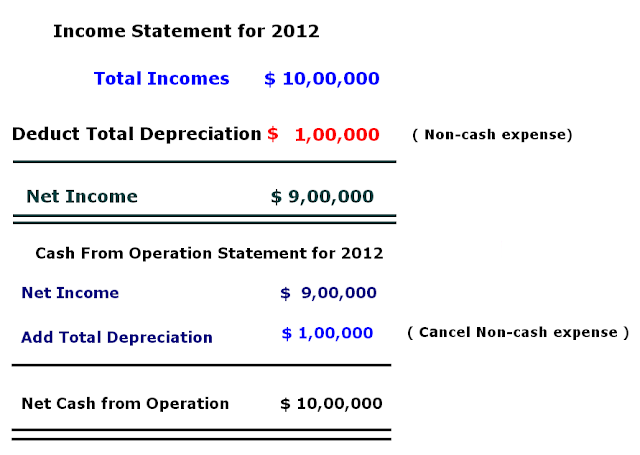Depreciation is added to net income to determine cash flow from operations because 1) Depreciation is not cash expense 2 ) We have already shown it as non-cash expense in the debited side of profit and loss account.

Now, I explain above two reasons one by one.

1. Depreciation is Non-Cash Expense

When we charge the depreciation, we do not pay the outside part for reducing our current liability. Depreciation on fixed asset is a non-cash expense or loss. We reduce the value of asset in the balance sheet. For showing our net profit correctly, we show it in the debit side of profit and loss account. But Cash flow from operation is different from net income. It is still net income but in the form of cash. We show all the incomes which we receive in the form of cash in Cash Flow from Operation Statement. We show all the expenses which we pay in the form of cash in Cash Flow from Operation Statement. After comparing both, we calculate the net cash flow from operation. So, it is necessary to add depreciation to net income to determine the net cash flow from operation.

2. We have already shown it as non-cash expense in the debited side of profit and loss account

By adding depreciation in net income, we cancel already deducted depreciation from total income. Let me explain from following screenshot and simple video tutorial..

## \$type=three\$a=hide\$cm=hide\$s=hide\$show=/2019/06/10-steps-to-become-better-investor.html\$l=hide

Name

false
ltr
item
Accounting Education: Why is Depreciation Added to Net Income to Determine Cash Flow from Operations
Why is Depreciation Added to Net Income to Determine Cash Flow from Operations
http://2.bp.blogspot.com/-oTnpKXDN1Js/UYtI8bTywII/AAAAAAAALX0/3APLLWxQgrA/s640/cancel+depreciation.PNG
http://2.bp.blogspot.com/-oTnpKXDN1Js/UYtI8bTywII/AAAAAAAALX0/3APLLWxQgrA/s72-c/cancel+depreciation.PNG
Accounting Education